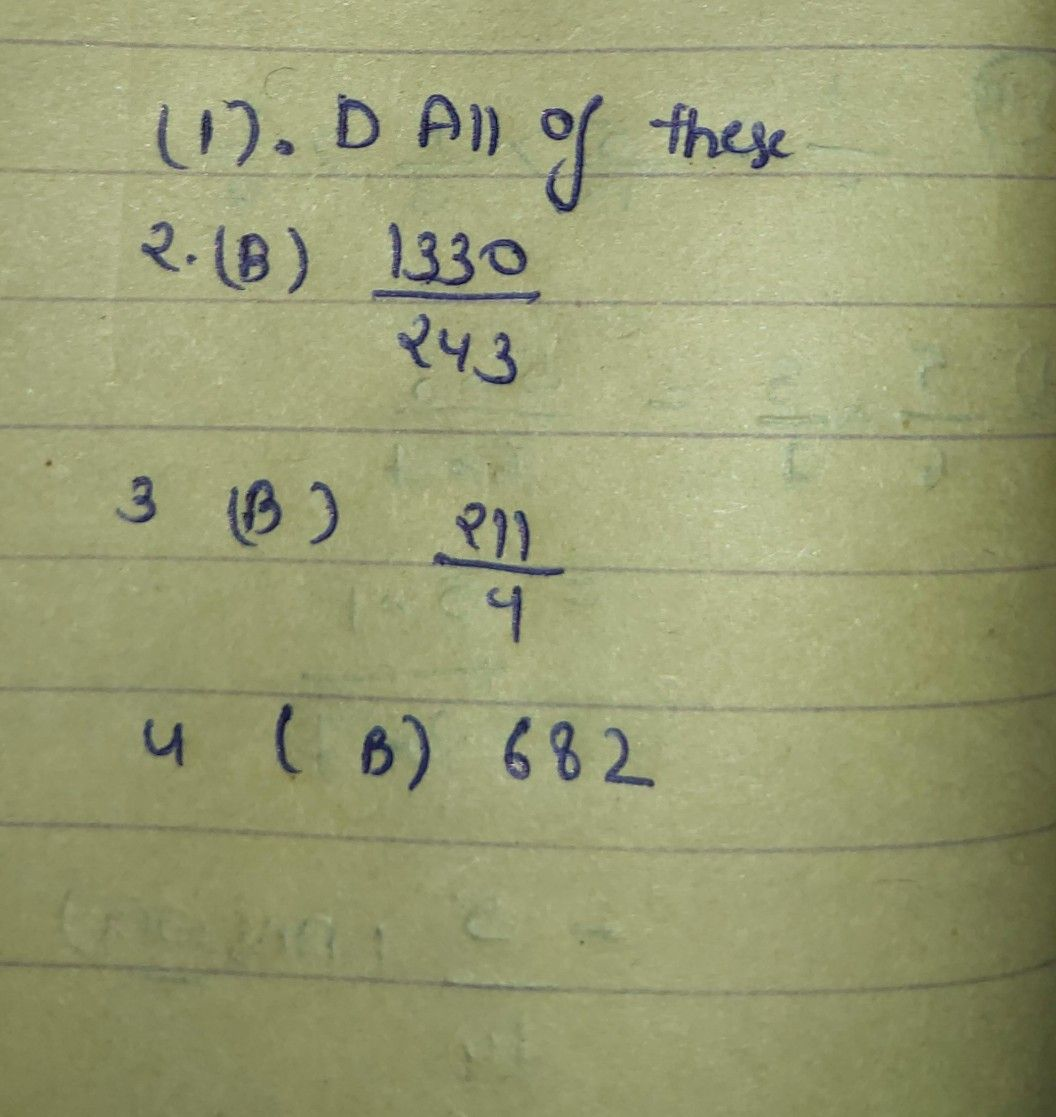Symbol
ProblemWhat I Know Multiple Choice. Read and analyze the following items and determine the letter of the correct answer from the given choices. Write your answer on a separate sheet of paper. 1. This refers to the sum of the terma of a geometric sequence. $.9$ Continuity A. Series D. All of these B. Limit $2$ Find the sum of the first six terms of a geometric sequence whose first term is 2 and common ratio $of\dfrac {2} {3}$ A. $1231$ C. $\dfrac {3990} {729}$ $7$ $\dfrac {760} {4551}$ B. $4567$ $\dfrac {1330} {243}$ D. $3.1$ Find the sum of the first five terms of the geometric sequence $4,6$ $9$ . A. $\dfrac {\dfrac {11} {2^{4}1}1} {4}$ $c.\dfrac {\dfrac {4} {11}4} {211}$ $D.$ B. $4.$ $32$ Find the sum of the first five terms of the geometric sequence $2,8,$ $128$ $243$ $682$ ... $C.6$ $679$ $A.$ $B.$ D. $743$
10th-13th grade
Geometry
Search count: 126
SolutionQanda teacher - Askhina